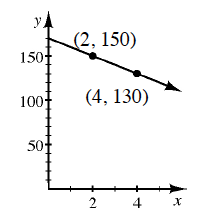### Home > GB8I > Chapter 5 Unit 6 > Lesson INT1: 5.2.1 > Problem5-53

5-53.

Draw a slope triangle and use it to write the equation of the line shown in the graph below. Homework Help ✎Use the same method you used in problem 5-48.

$y = −10x + 170$